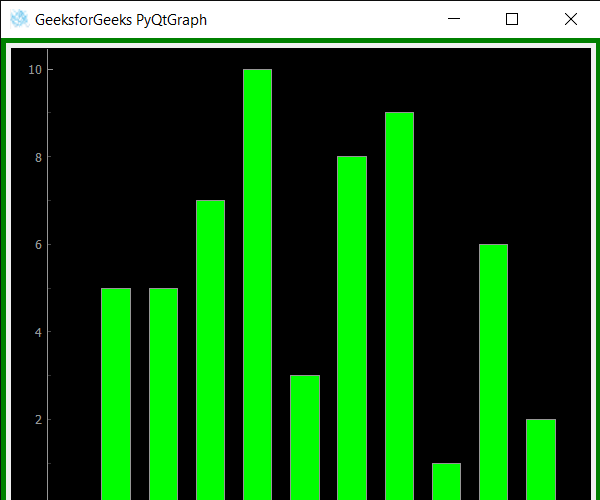# PyQtGraph – Setting Style Sheet of Plot Window

In this article we will see how we can set style sheet to the plot window in the PyQtGraph module. PyQtGraph is a graphics and user interface library for Python that provides functionality commonly required in designing and science applications. Its primary goals are to provide fast, interactive graphics for displaying data (plots, video, etc.) and second is to provide tools to aid in rapid application development (for example, property trees such as used in Qt Designer).Plot windows consist of two main parts: the Plot Panel containing the actual plotted graphics and the Control Panel. Style sheet is the sheet which define the visual layout of the window for example border, color etc.

We can create a plot window with the help of command given below

```# creating a pyqtgraph plot window
window = pg.plot()
```

In order to do this we use `setStyleSheet` method with the plot window object

Syntax : window.setStyleSheet(sheet)

Argument : It takes string as argument

Return : It returns None

Below is the implementation

 `# importing QtGui to use QIcon ` `from` `PyQt5.QtGui ``import` `*`  ` `  `# importing pyqtgraph as pg ` `import` `pyqtgraph as pg ` ` `  `# importing QtCore and QtGui from the pyqtgraph module ` `from` `pyqtgraph.Qt ``import` `QtCore, QtGui ` ` `  `# importing numpy as np ` `import` `numpy as np ` ` `  `import` `time ` ` `  `# creating a pyqtgraph plot window ` `window ``=` `pg.plot() ` ` `  `# icon for plot window ` `icon ``=` `QIcon(``"logo.png"``) ` ` `  `# setting icon to the plot window ` `window.setWindowIcon(icon) ` ` `  `# setting window geometry ` `# left = 100, top = 100 ` `# width = 600, height = 500 ` `window.setGeometry(``100``, ``100``, ``600``, ``500``) ` ` `  `# title for the plot window ` `title ``=` `"GeeksforGeeks PyQtGraph"` ` `  `# setting window title to plot window ` `window.setWindowTitle(title) ` ` `  `# create list for y-axis ` `y1 ``=` `[``5``, ``5``, ``7``, ``10``, ``3``, ``8``, ``9``, ``1``, ``6``, ``2``] ` ` `  `# create horizontal list i.e x-axis ` `x ``=` `[``1``, ``2``, ``3``, ``4``, ``5``, ``6``, ``7``, ``8``, ``9``, ``10``] ` ` `  `# create pyqt5graph bar graph item  ` `# with width = 0.6 ` `# with bar colors = green ` `bargraph1 ``=` `pg.BarGraphItem(x ``=` `x, height ``=` `y1, width ``=` `0.6``, brush ``=``'g'``) ` ` `  `# add item to plot window ` `# adding bargraph item to the window ` `window.addItem(bargraph1) ` ` `  `# setting style sheet to the plot window ` `window.setStyleSheet(``"border : 5px solid green;"` `                     ``"padding : 5px;"``) ` ` `  ` `  ` `  `# main method ` `if` `__name__ ``=``=` `'__main__'``: ` `     `  `    ``# importing system ` `    ``import` `sys ` `     `  `    ``# Start Qt event loop unless running in interactive mode or using  ` `    ``if` `(sys.flags.interactive !``=` `1``) ``or` `not` `hasattr``(QtCore, ``'PYQT_VERSION'``): ` `        ``QtGui.QApplication.instance().exec_() ` `        `

Output :Attention geek! Strengthen your foundations with the Python Programming Foundation Course and learn the basics.

To begin with, your interview preparations Enhance your Data Structures concepts with the Python DS Course.

My Personal Notes arrow_drop_upCheck out this Author's contributed articles.

If you like GeeksforGeeks and would like to contribute, you can also write an article using contribute.geeksforgeeks.org or mail your article to contribute@geeksforgeeks.org. See your article appearing on the GeeksforGeeks main page and help other Geeks.

Please Improve this article if you find anything incorrect by clicking on the "Improve Article" button below.

Article Tags :

Be the First to upvote.

Please write to us at contribute@geeksforgeeks.org to report any issue with the above content.